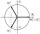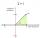Linear imaginary equation

Given that"this is z star"
Find the value of the complex number z.

Result

z = (Correct answer is: -i)Solution:Leave us a comment of example and its solution (i.e. if it is still somewhat unclear...):Be the first to comment!To solve this verbal math problem are needed these knowledge from mathematics:

Do you have a linear equation or system of equations and looking for its solution? Or do you have quadratic equation? Try our complex numbers calculator.

Next similar examples:

1. Imaginary numbersFind two imaginary numbers whose sum is a real number. How are the two imaginary numbers related? What is its sum?
2. Ball gameRichard, Denis and Denise together scored 932 goals. Denis scored 4 goals over Denise but Denis scored 24 goals less than Richard. Determine the number of goals for each player.
3. AsymptoteWhat is the vertical asymptote of ?
4. Solve 3Solve quadratic equation: (6n+1) (4n-1) = 3n2
5. Intercept with axisF(x)=log(x+4)-2, what is the x intercept
6. Insert into GPBetween numbers 5 and 640 insert as many numbers to form geometric progression so sum of the numbers you entered will be 630. How many numbers you must insert?
7. Geometric sequence 5About members of geometric sequence we know: ? ? Calculate a1 (first member) and q (common ratio or q-coefficient)
8. AS sequenceIn an arithmetic sequence is given the difference d = -3 and a71 = 455. a) Determine the value of a62 b) Determine the sum of 71 members.
9. De Moivre's formulaThere are two distinct complex numbers z such that z3 is equal to 1 and z is not equal 1. Calculate the sum of these two numbers.
10. Median and modusRadka made 50 throws with a dice. The table saw fit individual dice's wall frequency: Wall Number: 1 2 3 4 5 6 frequency: 8 7 5 11 6 13 Calculate the modus and median of the wall numbers that Radka fell.
11. Theorem proveWe want to prove the sentence: If the natural number n is divisible by six, then n is divisible by three. From what assumption we started?
12. Complex number coordinatesWhich coordinates show the location of -2+3i
13. ReciprocalCalculate reciprocal of z=0.8-1.8i:
14. Is complexAre these numbers 2i, 4i, 2i + 1, 8i, 2i + 3, 4 + 7i, 8i, 8i + 4, 5i, 6i, 3i complex?
15. Im>0?Is -10i a positive number?
16. Geometric progression 2There is geometric sequence with a1=5.7 and quotient q=-2.5. Calculate a17.
17. Calculation of CNCalculate: ?